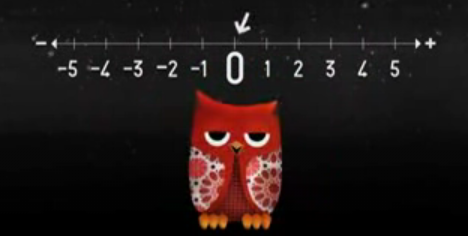# Sunday song: Zeros

The Ladybug and I have been listening to Here Come the 123s, a CD that combines two of my favorite things: They Might Be Giants and mathematics.   Here is another song off the album called “Zeros,” which describes the many roles played by the number 0, both as a place-holder in a positional number system, and as an integer itself.   (Of course, the definition posited in the song for the integer 0 is purely tautological, but hey…)   Here’s the video on YouTube, with some jokes.## Zeros

Zero! Zeros! Zeros mean so much.
Zero! Zeros! Zeros mean so much,
In numbers big (one million)
To small (one millionth)
And a number that means nothing at all (the number zero).

Zero! Zeros! Zeros mean so much.
Zero! Zeros! Zeros mean so much,
In numbers big (ten, one hundred, one thousand)
And numbers  small (one tenth, one one-hundredth, one one-thousandth)
And a number that means nothing at all (the number zero).

One definition of zero is the mathematical value between positive and  negative values.

Zero! Zeros! Zeros mean so much.
Zero! Zeros! Zeros mean so much,
In numbers big (one million)
To small (one millionth)
And a number that means nothing at all (the number zero).

This entry was posted in nerdify. Bookmark the permalink.